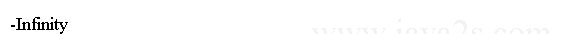# Java double type

In this chapter you will learn:

### double type

double represents double-precision numbers. double is 64-bit width and its range is from 4.9e-324 to 1.8e+308 approximately.

Here is a program that uses double variables to compute the area of a circle:

``````public class Main {
public static void main(String args[]) {
double pi, r, a;
/*from   ja v a  2  s  .  com*/
r = 10.8888; // radius of circle
pi = 3.1415926; // pi, approximately
a = pi * r * r;

System.out.println("Area of circle is " + a);
}
}
``````

The output:### double type Literals

double type numbers have decimal values with a fractional component. They can be expressed in either standard or scientific notation. Standard notation consists of a whole number component followed by a decimal point followed by a fractional component. For example, `2.0`, `3.14159`, and `0.6667`.

``````public class Main {
public static void main(String args[]) {
double d =  3.14159;
System.out.print(d);//3.14159
} //from   ja v a2 s  .c o  m
}
``````

Scientific notation uses a standard-notation, floating-point number plus a suffix that specifies a power of `10` by which the number is to be multiplied. The exponent is indicated by an E or e followed by a decimal number, which can be positive or negative. For example, `6.02E23`, `314159E-05`, and `4e+100`.

``````public class Main {
public static void main(String[] argv) {
double d1 = 6.022E23;
double d2 = 314159E-05;
double d3 = 2e+100;
//from  j ava  2s.  c  om
System.out.println("d1 is " + d1);
System.out.println("d2 is " + d2);
System.out.println("d3 is " + d3);
}

}
``````

The output generated by this program is shown here:You can explicitly specify a double literal by appending a D or d.

``````public class Main {
public static void main(String args[]) {
double d =  3.14159D;
System.out.print(d);//3.14159
} /*  ja v  a2 s  . com*/
}
``````

Java's floating-point calculations are capable of returning `+infinity`, `-infinity`, `+0.0`, `-0.0`, and `NaN`

dividing a positive number by `0.0` returns `+infinity`. For example, `System.out.println(1.0/0.0);` outputs Infinity.

``````public class Main{
public static void main(String[] args) {
System.out.println(1.0/0.0);/*  java  2s  .  c o  m*/
}

}
``````

### double Infinity

Dividing a negative number by `0.0` outputs `-infinity`. For example, `System.out.println(-1.0/0.0);` outputs `-Infinity`.

``````public class Main{
public static void main(String[] args) {
System.out.println(-1.0/0.0);// j  a v  a  2s  .c om
}

}
``````

Output:#### Next chapter...

What you will learn in the next chapter:

1. What is double type NaN(Not a Number)
Home » Java Tutorial » Primitive Data Types